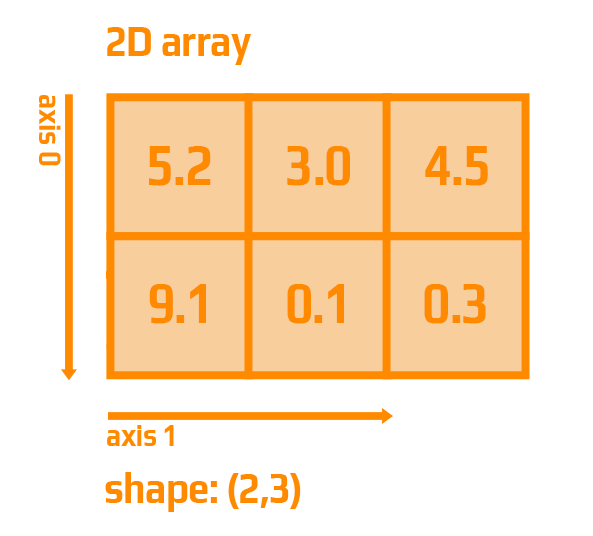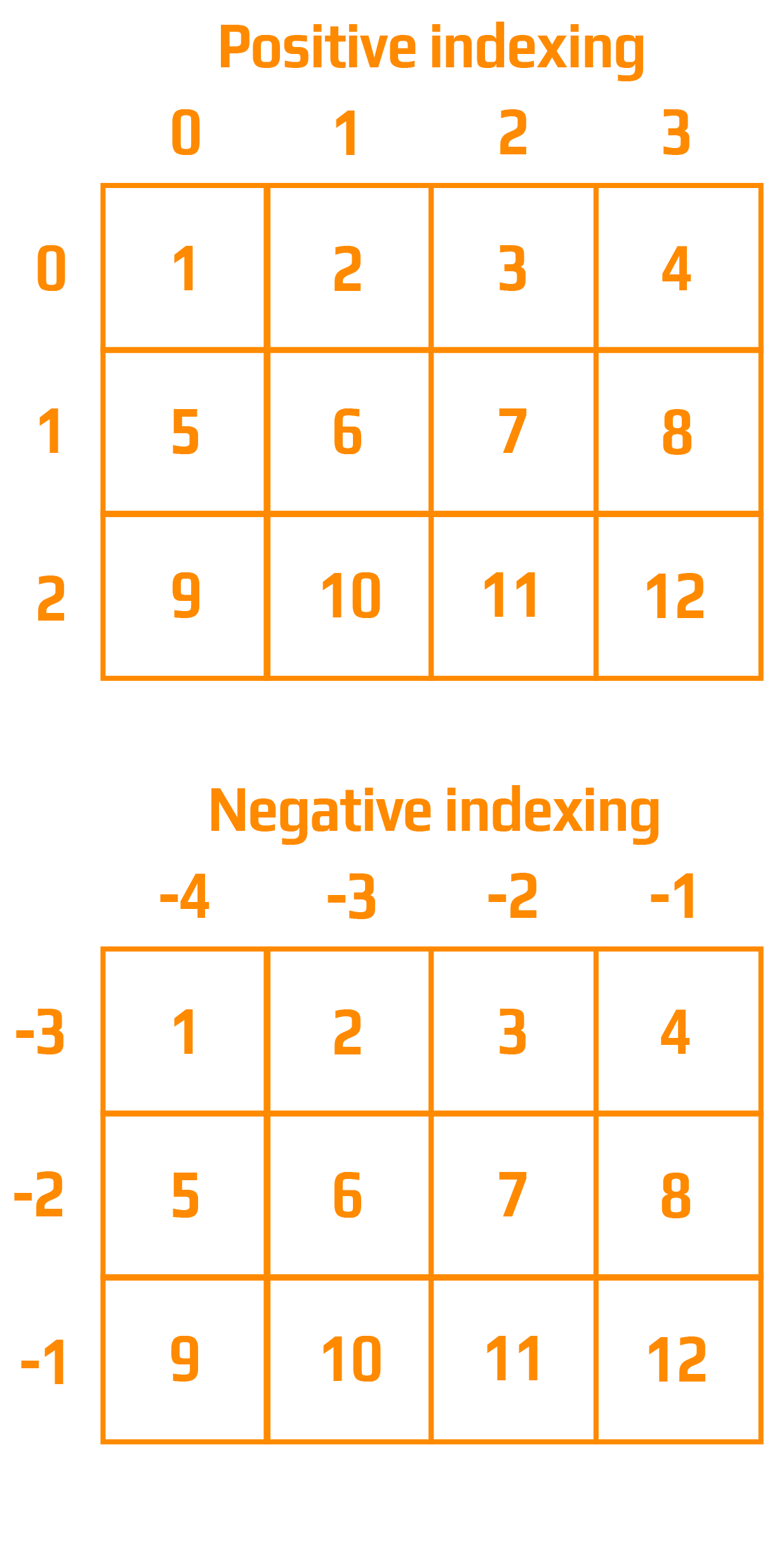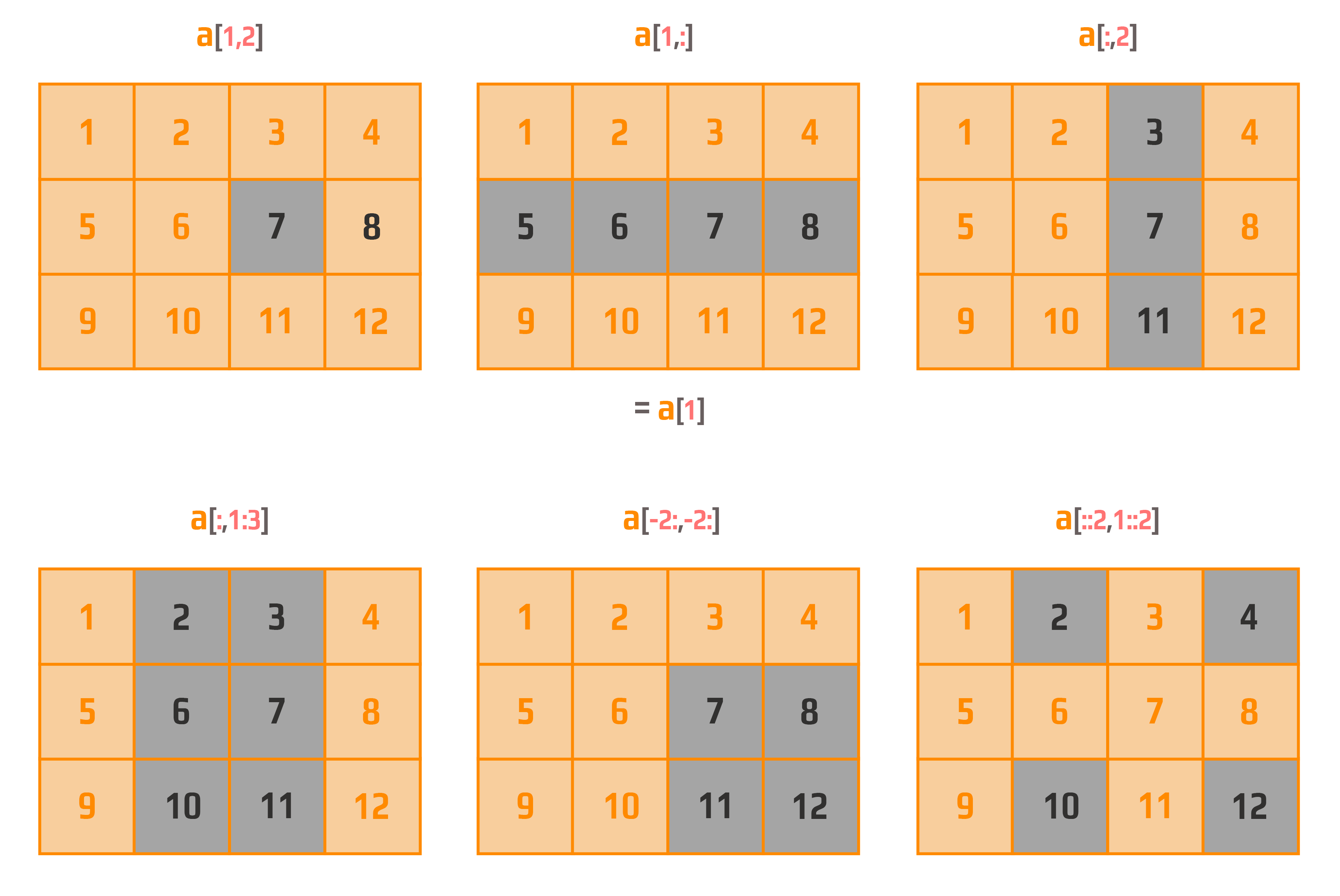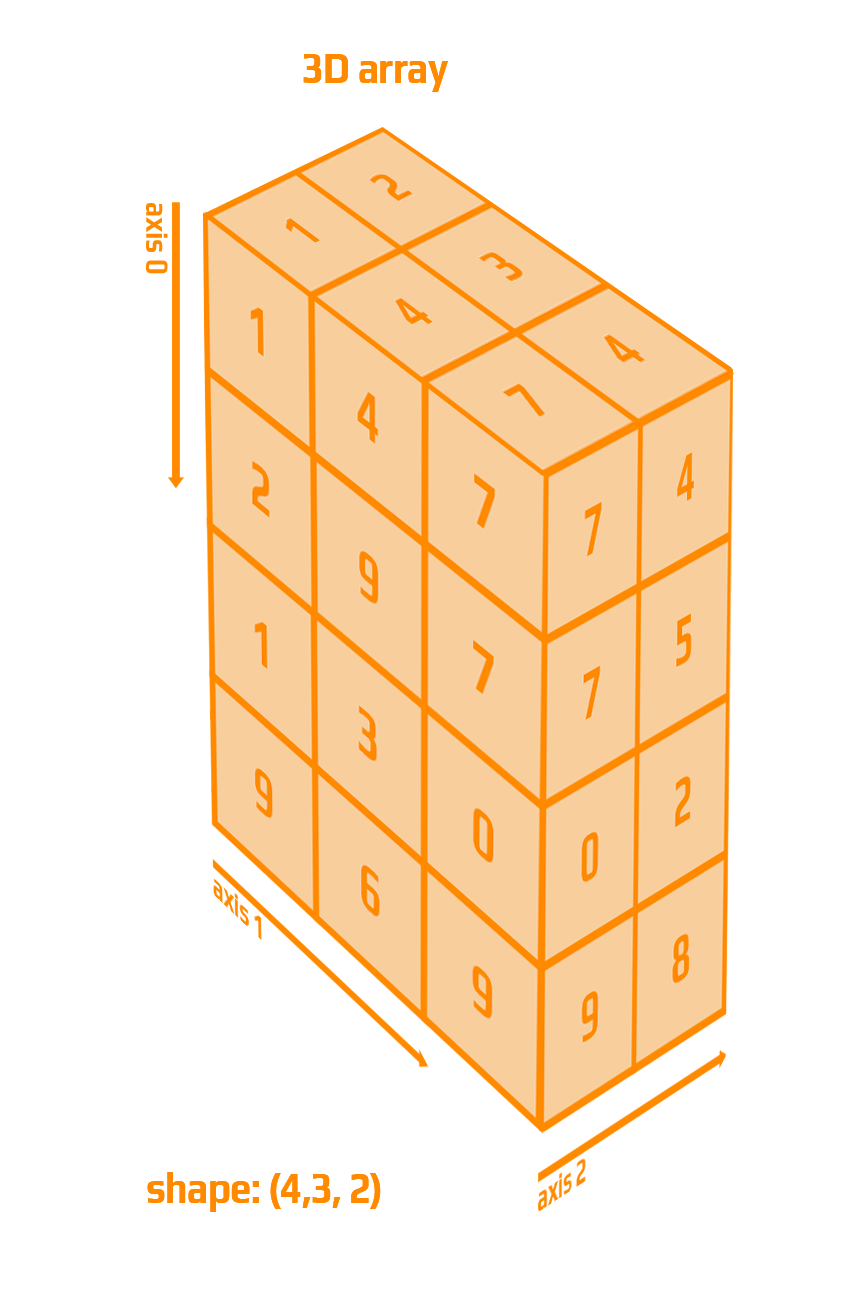Course Content

# NumPy in a Nutshell

NumPy in a Nutshell

##Access 2-D and 3-D Arrays

Let's have a look at an example of a 2-D array with axis numbering:Let's have a look at an example of indexing (both positive and negative) in 2-D arrays:Let's examine the syntax of slicing: `array[start_row: end_row: step_row, start_column: end_column: step_column]`, where:

• start_row is the index from which row slicing begins;
• end_row is the index where row slicing stops (note that this index is not included);
• step_row is the parameter that specifies the intervals between row indices;
• start_column is the index from which column slicing starts;
• end_column is the index where column slicing ends (note that this index is not included);
• step_column is the parameter that determines the intervals between column indices.

Now, let's refer to the following image:Let's have a look at an example of a 3-D array with axis numbering:Consider the following array: `[[6, 5, 7, 8], [65, 2, 7, 9]]`.
1. Retrieve the fourth element from the first part of the array `[6, 5, 7, 8]`, and the first element from the second part of the array `[65, 2, 7, 9]`.